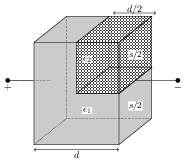# Parallel Plate Capacitor with and without Dielectrics

## Problems from IIT JEE

Problem (IIT JEE 2015):A parallel plate capacitor having plates of area $s$ and plate separation $d$, has capacitance $C_1$ in air. When two dielectrics of different relative permittivities ($\epsilon_1=2$ and $\epsilon_2=4$) are introduced between the two plates as shown in the figure, the capacitance becomes $C_2$. The ratio $C_2/C_1$ is,

1. 6/5
2. 5/3
3. 7/5
4. 7/3

Solution: The capacitance of an air filled parallel plate capacitor having plates of area $s$ and plate separation $d$ is given by $C_1=\epsilon_0 s/d$. Consider the case when two dielectrics of relative permittivities ($\epsilon_1=2$ and $\epsilon_2=4$) are introduced between the two plates as shown in the figure. We can think of this capacitor to be made up of three parts:

1. Capacitor of plate area $s/2$, plate separation $d$, and filled with a dielectric of relative permittivity $\epsilon_1=2$ (lower half). The capacitance of this part is $C_a=\epsilon_1 \epsilon_0\frac{ s/2}{d}=\epsilon_0 \frac{s}{d}=C_1$.
2. Capacitor of plate area $s/2$, plate separation $d/2$, and filled with a dielectric of relative permittivity $\epsilon_1=2$ (left upper half). The capacitance of this part is $C_b=\epsilon_1 \epsilon_0 \frac{s/2}{d/2}=2\epsilon_0 \frac{s}{d}=2C_1$.
3. Capacitor of plate area $s/2$, plate separation $d/2$, and filled with a dielectric of relative permittivity $\epsilon_2=4$ (right upper half). The capacitance of this part is $C_c=\epsilon_2 \epsilon_0 \frac{s/2}{d/2}=4\epsilon_0 \frac{s}{d}=4C_1$.
The capacitors $C_b$ and $C_c$ are in series with equivalent capacitance, \begin{align} C_{bc}=\frac{C_b C_c}{C_b+C_c}=\frac{(2C_1)(4C_1)}{(2C_1)+(4C_1)}=\frac{4}{3}C_1.\nonumber \end{align} The capacitor $C_{bc}$ is in parallel with $C_a$. The equivalent capacitance is, \begin{align} C_2=C_{abc}=C_{a}\parallel C_{bc}=C_1+\frac{4}{3}C_1=\frac{7}{3}C_1.\nonumber \end{align}